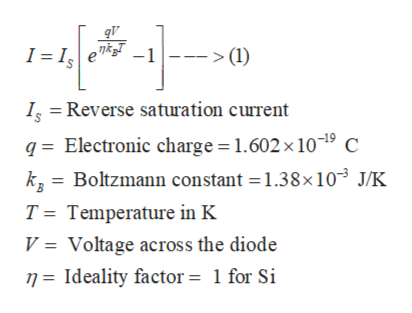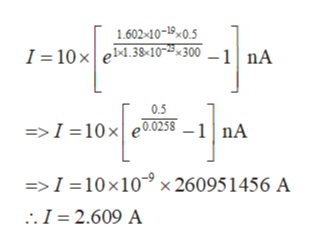# For a silicon pn junction under forward biased condition, the reverse saturation current is 10 nA. If the value of the forward voltage across the diode is 0.5 V and the observation is being made at 300 K, then what will be the value of the diode current?

Question
2 views

For a silicon pn junction under forward biased condition, the reverse saturation current is 10 nA. If the value of the forward voltage across the diode is 0.5 V and the observation is being made at 300 K, then what will be the value of the diode current?

check_circle

Step 1

Using Shockley’s equation, the value of the current flowing through a p-n junction diode can be expressed ashelp_outlineImage Transcriptioncloseqv -1 >(1) I I I Reverse saturation current q Electronic charge 1.602 x 10-19 C k3 Boltzmann constant 1.38x10 J/K T Temperature in K Voltage across the diode V n=Ideality factor = 1 for Si fullscreen
Step 2

In this case, the values of the reverse saturation current, temperature and the voltage across the diode are given as

Step 3

So, using equation (1), the value of the current through the diode will be

...help_outlineImage Transcriptionclose1.602-10-190.5 I 10xe4.38«10"~*«300 _1nA 0.5 =>I =10 x| e0.0258 1 nA =>I = 10 x10 x 260951456 A .I 2.609 A fullscreen

### Want to see the full answer?

See Solution

#### Want to see this answer and more?

Solutions are written by subject experts who are available 24/7. Questions are typically answered within 1 hour.*

See Solution
*Response times may vary by subject and question.
Tagged in

### Electrical Engineering